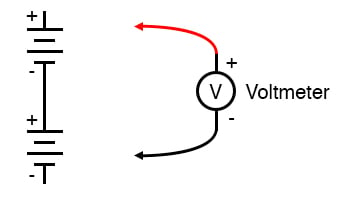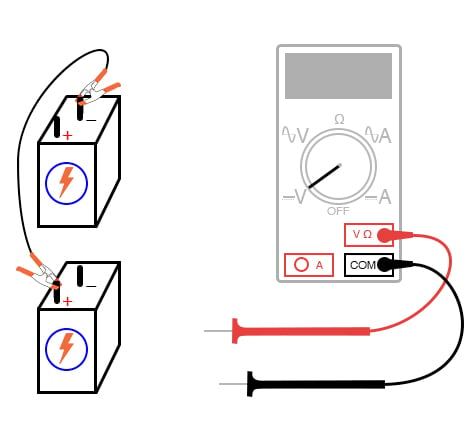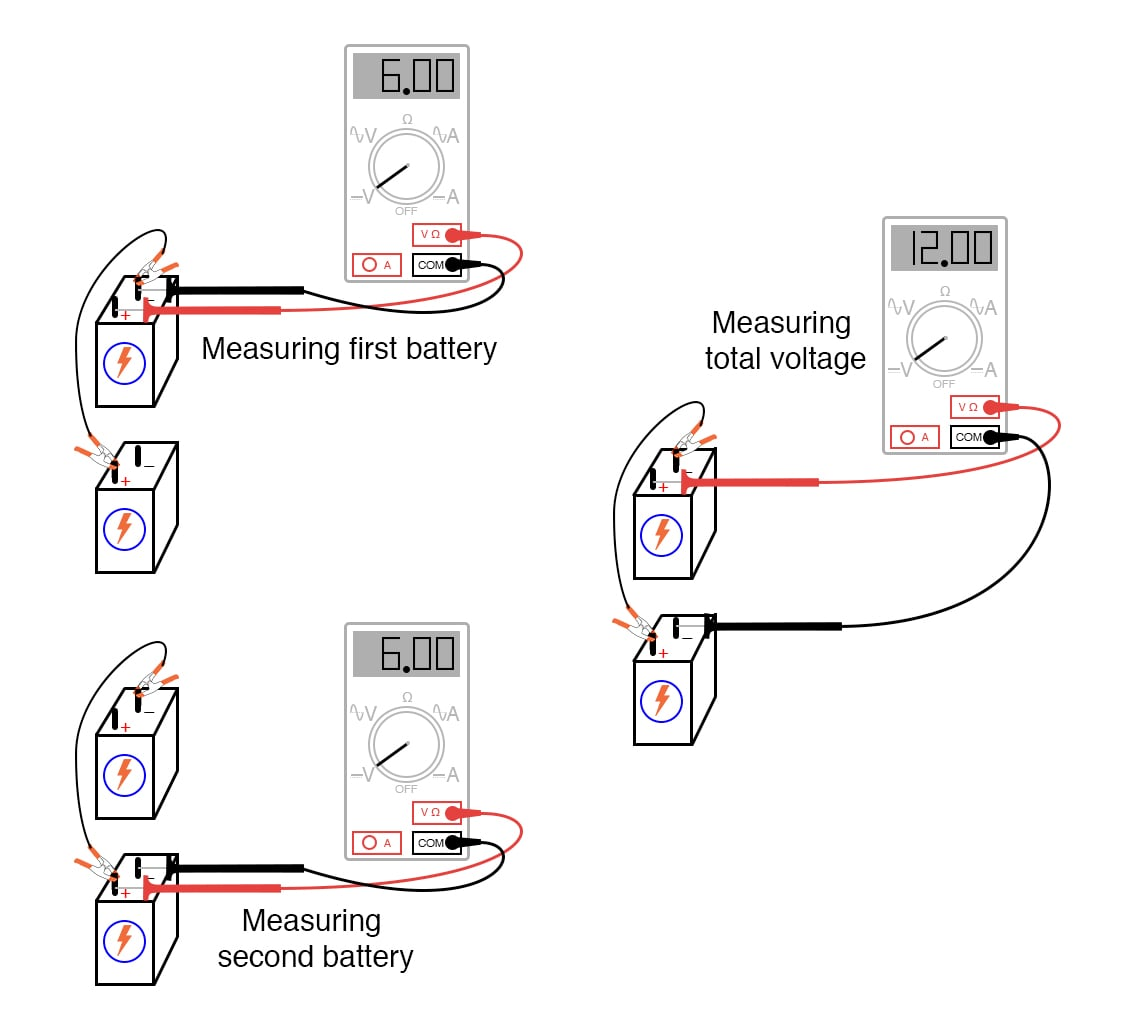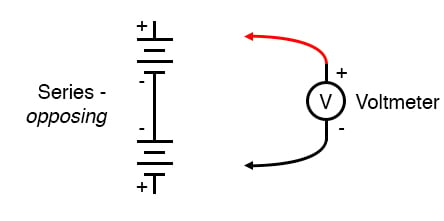# Series Batteries

## Chapter 3 - DC Circuits

PARTS AND MATERIALS

• Two 6-volt batteries
• One 9-volt battery

Actually, any size batteries will suffice for this experiment, but it is recommended to have at least two different voltages available to make it more interesting.

CROSS-REFERENCES

Lessons In Electric Circuits, Volume 1, chapter 5: “Series and Parallel Circuits”

Lessons In Electric Circuits, Volume 1, chapter 11: “Batteries and Power Systems”

LEARNING OBJECTIVES

• To illustrate on how to connect batteries to obtain varying voltages.

SCHEMATIC DIAGRAMILLUSTRATIONINSTRUCTIONS

Connecting components in series means to connect them in-line with each other, so that there is but a single path for electrons to flow through them all.

If you connect batteries so that the positive of one connects to the negative of the other, you will find that their respective voltages add.

Measure the voltage across each battery individually as they are connected, then measure the total voltage across them both, like this:Try connecting batteries of different sizes in series with each other, for instance a 6-volt battery with a 9-volt battery.

What is the total voltage in this case? Try reversing the terminal connections of just one of these batteries, so that they are opposing each other like this:How does the total voltage compare in this situation to the previous one with both batteries “aiding?”

Note the polarity of the total voltage as indicated by the voltmeter indication and test probe orientation.

Remember, if the meter’s digital indication is a positive number, the red probe is positive (+) and the black probe negative (-); if the indication is a negative number, the polarity is “backward” (red=negative, black=positive).

Analog meters simply will not read properly if reverse-connected, because the needle tries to move the wrong direction (left instead of right).

Can you predict what the overall voltage polarity will be, knowing the polarities of the individual batteries and their respective strengths?

RELATED WORKSHEETS:

• Share
Published under the terms and conditions of the Design Science License